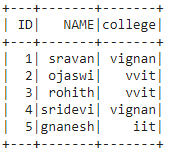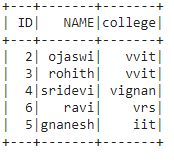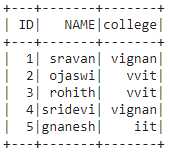# Drop rows containing specific value in PySpark dataframe

• Last Updated : 30 Jun, 2021

In this article, we are going to drop the rows with a specific value in pyspark dataframe.

Creating dataframe for demonstration:

## Python3

 `# importing module``import` `pyspark`` ` `# importing sparksession from pyspark.sql module``from` `pyspark.sql ``import` `SparkSession`` ` `# creating sparksession and giving an app name``spark ``=` `SparkSession.builder.appName(``'sparkdf'``).getOrCreate()`` ` `# list  of students  data``data ``=` `[[``"1"``, ``"sravan"``, ``"vignan"``],``        ``[``"2"``, ``"ojaswi"``, ``"vvit"``],``        ``[``"3"``, ``"rohith"``, ``"vvit"``],``        ``[``"4"``, ``"sridevi"``, ``"vignan"``],``        ``[``"6"``, ``"ravi"``, ``"vrs"``],``        ``[``"5"``, ``"gnanesh"``, ``"iit"``]]`` ` `# specify column names``columns ``=` `[``'ID'``, ``'NAME'``, ``'college'``]`` ` `# creating a dataframe from the lists of data``dataframe ``=` `spark.createDataFrame(data, columns)`` ` `print``(``'Actual data in dataframe'``)``dataframe.show()`

Output:Method 1: Using where() function

This function is used to check the condition and give the results. That means it drops the rows based on the values in the dataframe column

Syntax: dataframe.where(condition)

Example 1: Python program to drop rows with college = vrs.

## Python3

 `# drop rows with college vrs``dataframe.where(dataframe.college!``=``'vrs'``).show()`

Output:Example 2: Python program to drop rows with ID=1

## Python3

 `# drop rows with id=1 ``dataframe.where(dataframe.``ID` `!``=``'1'``).show()`

Output:Method 2: Using filter() function

This function is used to check the condition and give the results, Which means it drops the rows based on the values in the dataframe column. Both are similar.

Syntax: dataframe.filter(condition)

Example: Python code to drop row with name = ravi.

## Python3

 `# drop rows with name = ravi ``dataframe.``filter``(dataframe.NAME !``=``'ravi'``).show()`

Output:My Personal Notes arrow_drop_up